# Egison Presentation for the Scheme and Functional Programming Workshop 2017

Sep 16, 2017

In the Scheme and Functional Programming Workshop 2017, I made a presentation on a new feature of Egison. The following are my slides and script. We can download my paper from arXiv.

### #1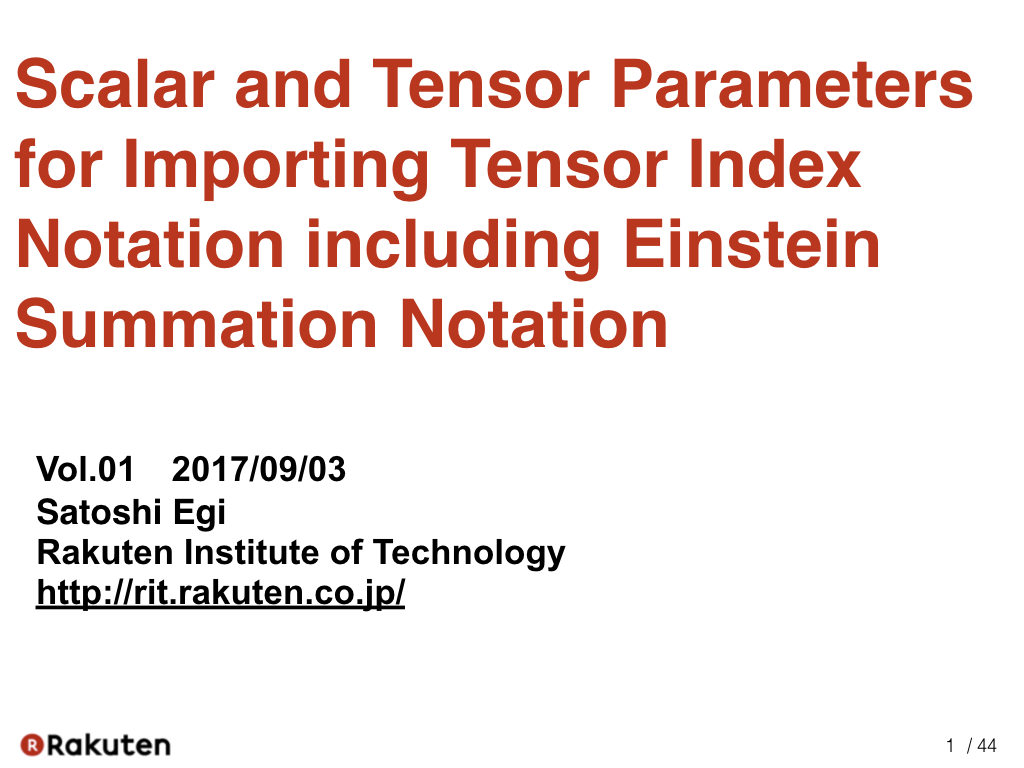Hello everybody. I am happy to be here. Thank you for inviting me. Today, I’d like talk about my method for importing tensor index notation including Einstein summation notation into programming languages.

### #2Currently, the proposed method is implemented in the Egison programming language. Egison is the programming language that I have been developing since 2011. I am implementing it in Haskell. We can install it using the “cabal install” command.

### #3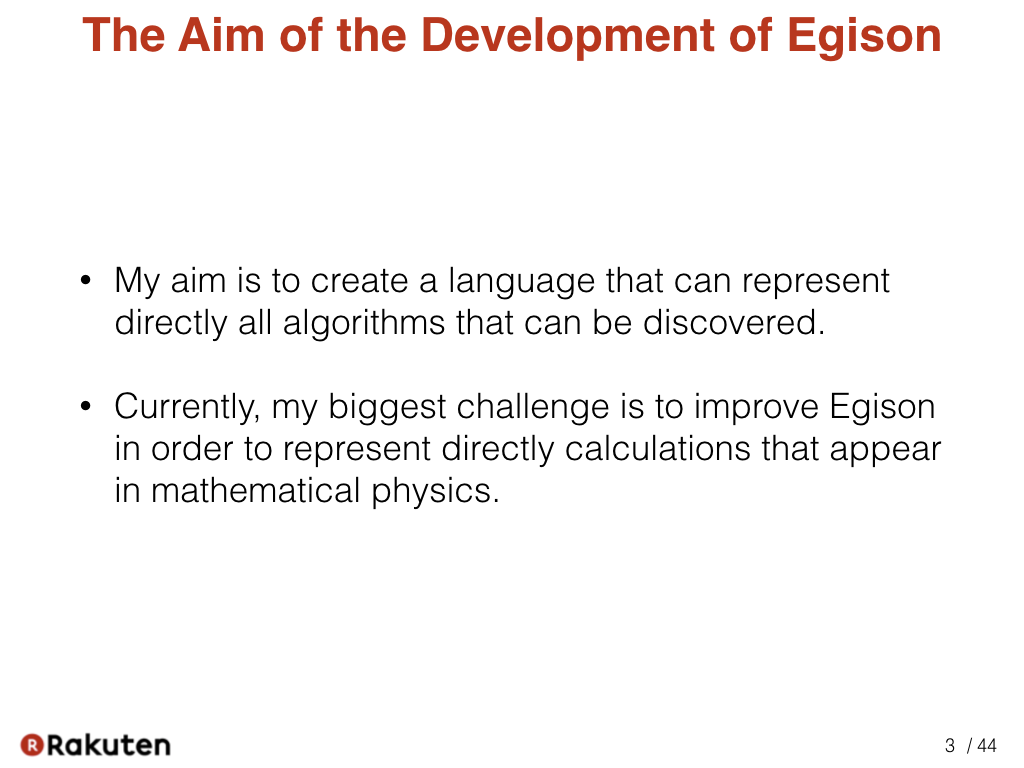The aim of the development of Egison is to create a language that can represent directly all algorithms that can be discovered and it’s a very big goal. Currently, my biggest challenge is to improve Egison in order to represent directly calculations that appear in mathematical physics.

### #4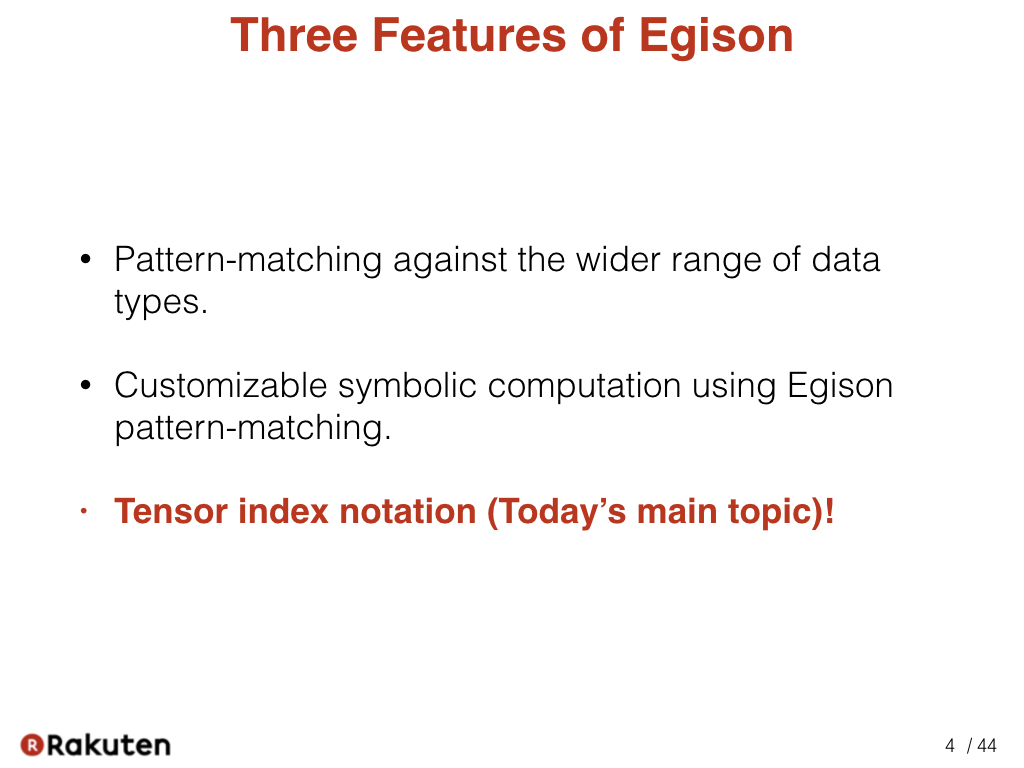The current version of Egison has three special features.

First, it has a strong pattern-matching facility. We can describe pattern-matching against the wider range of data than the popular functional languages.

Second, it support symbolic computation as Mathematica. It is implemented utilizing Egison pattern-matching.

Third, it supports tensor index notation. This is today’s topic.

### #5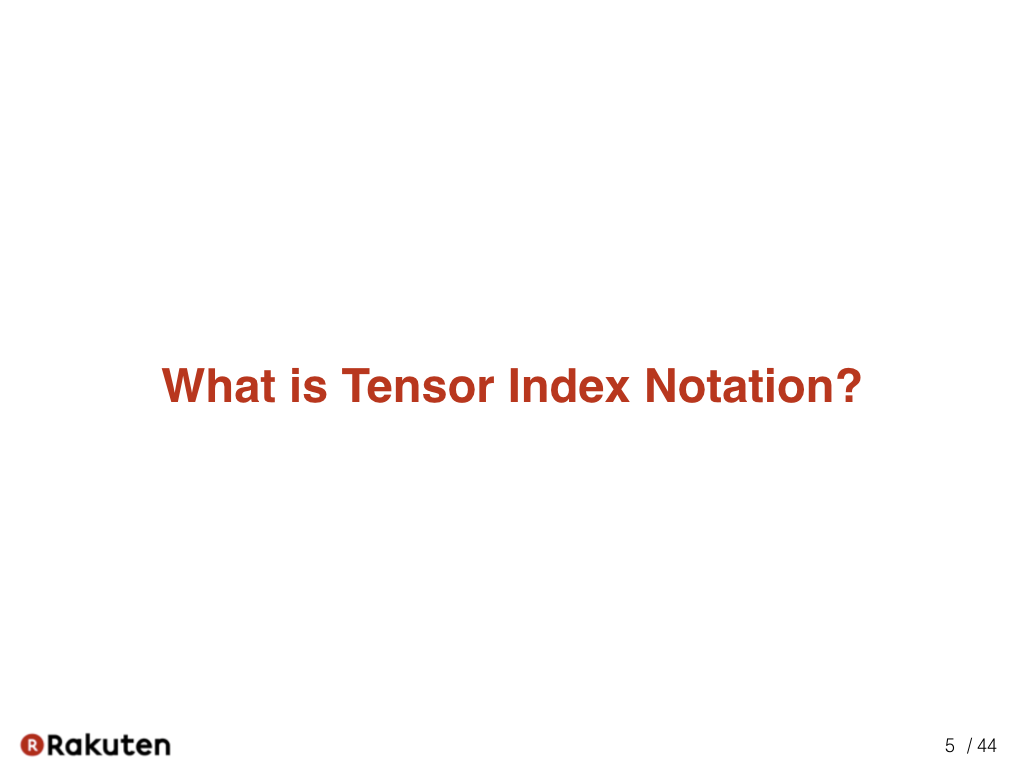Now, let me show what is tensor index notation.

### #6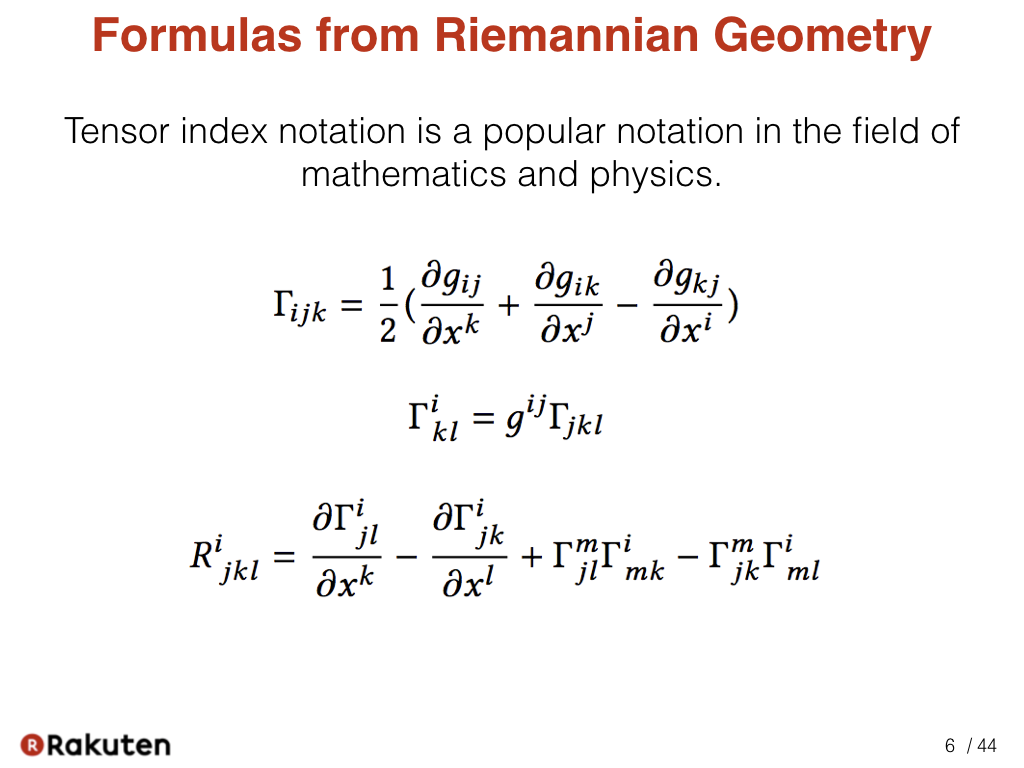Tensor index notation is a popular notation in the field of mathematics and physics. Here are formulas from Riemannian geometry. We can see many indices are used in the formulas.

### #7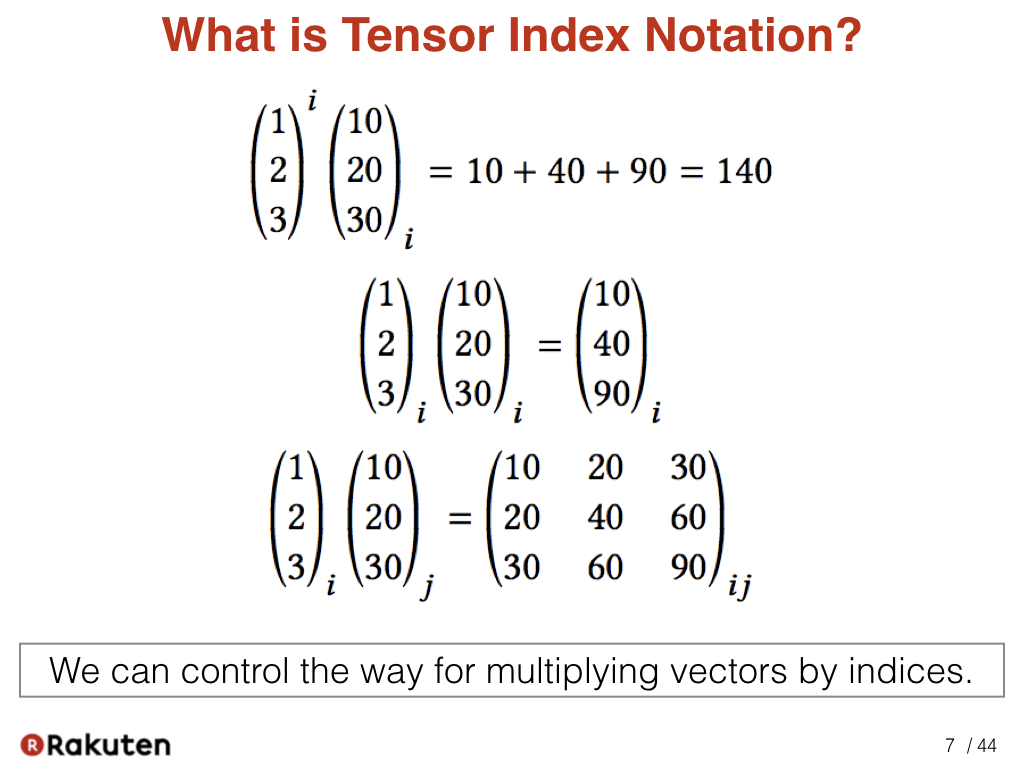These are the simplest samples of tensor index notation. We represent inner-product, Hadamard product, and tensor-product of two vectors by just changing the index. In index notation, the position of the index has meaning. As the first sample, when the index is repeated in a superscript and a subscript, it contracts the components. That is, it calculate the inner-product. This rule is called Einstein summation convention. As the second sample, when the index is repeated in the same position, each component of vectors are multiplied. As the third sample, when the indices are different, the tensor-product of two vectors are calculated. That way, we can control the way of multiplying vectors.

### #8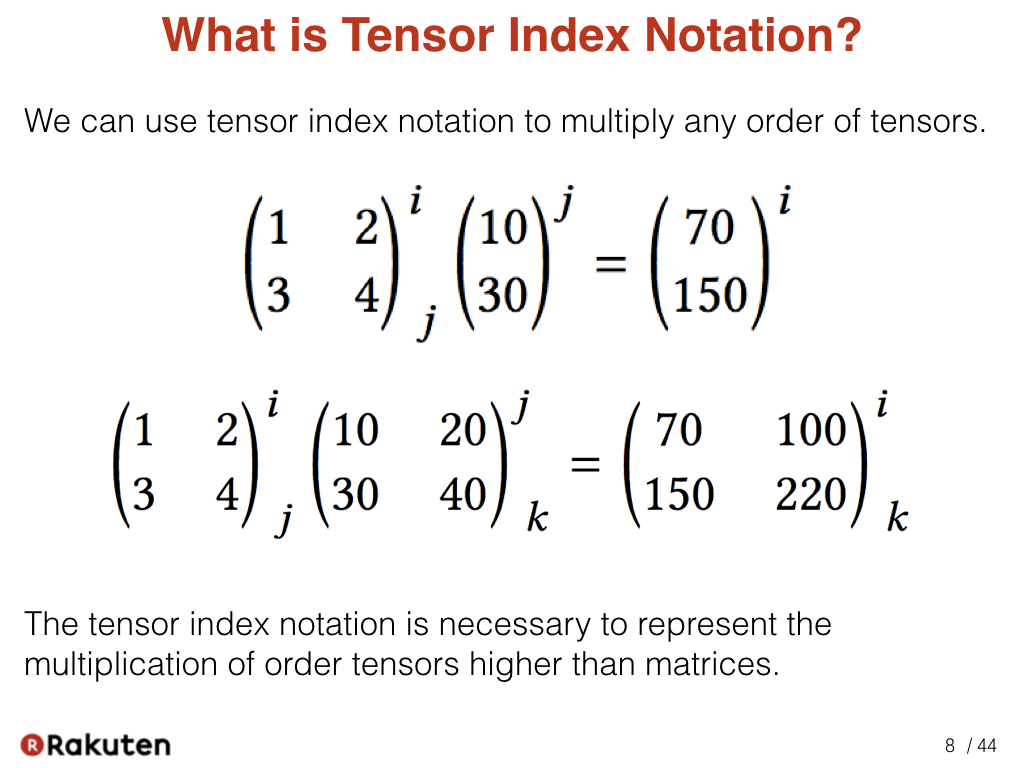We can append multiple indices to the high-order tensors. In this sample, we represent multiplication of matrix using index notation. Index notation is necessary to represent the multiplication of the higher-order tensors than matrices.

### #9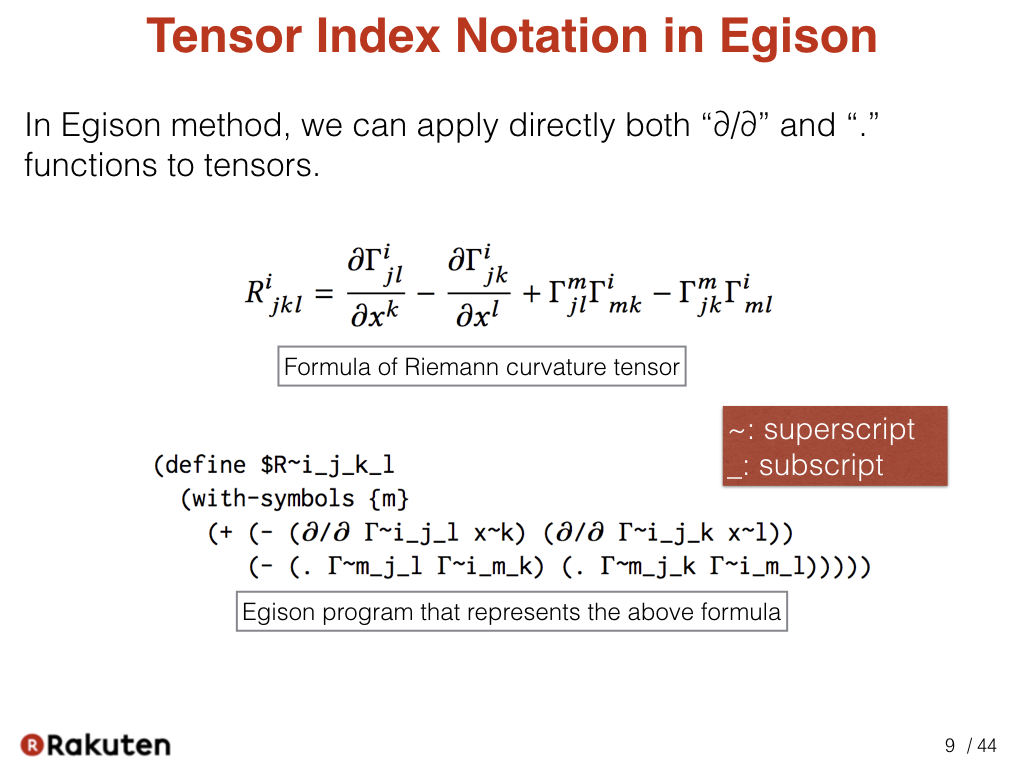This is a first sample of Egison using tensor index notation. The program below represent the above mathematical formula.

Please note that we can directly apply both of the “∂/∂” and “.” functions to tensors in Egison. This enables us to represent the above mathematical formula directly as a program. In Egison, we represent superscripts with the “~” sign and subscript with the “_” sign.

### #10Next, let me introduce the related work.

### #11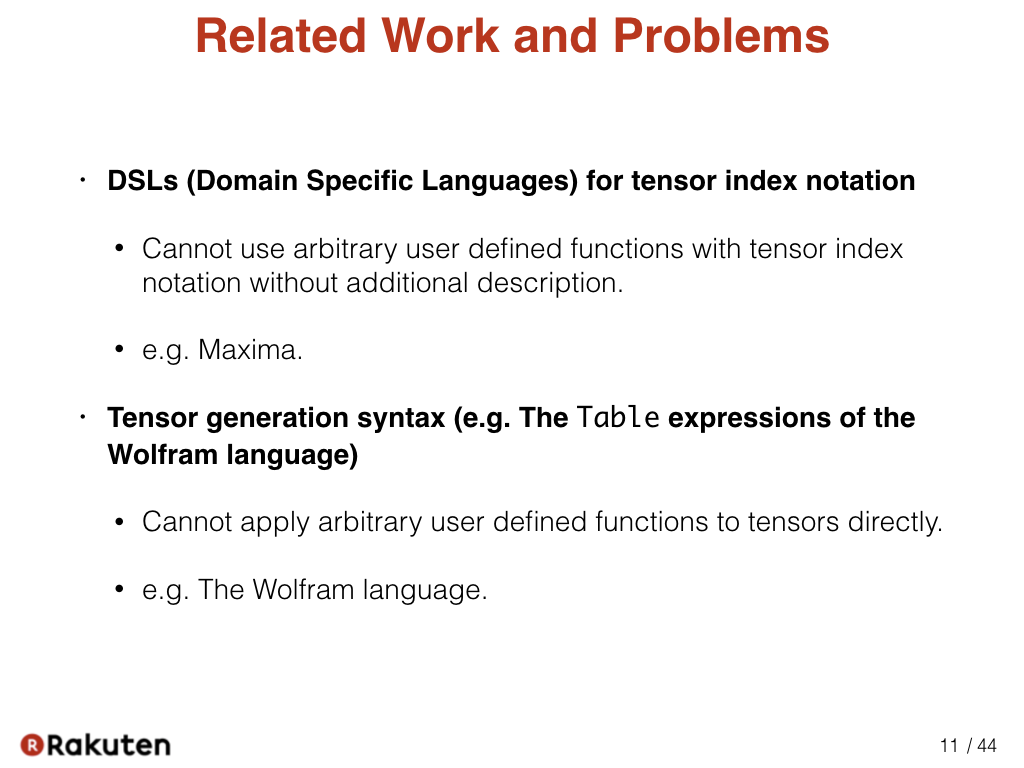There are two existing methods for using tensor index notation in programming. A method that introduces special operators supporting index notation and a method that introduces special syntax for index notation.

### #12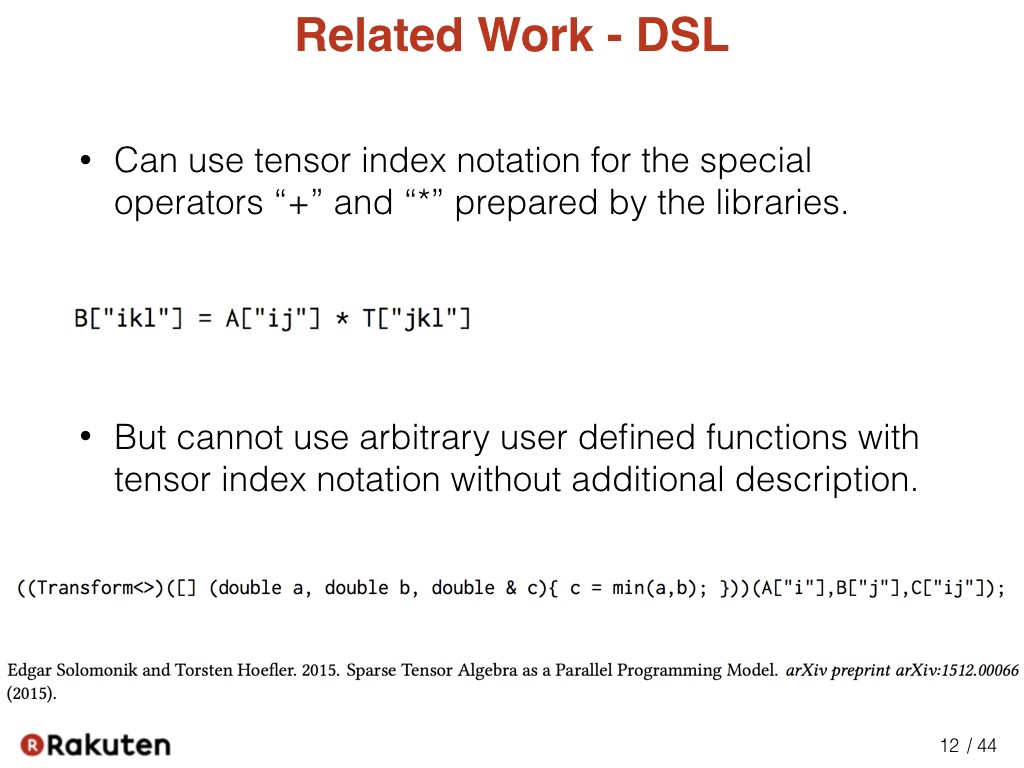Using the first method enables index notation to be represented directly in a program. However, this has the disadvantage that index notation can be used only by functions that are specially prepared to use it. It requires additional description to use arbitrary user defined functions with tensor index notation.

### #13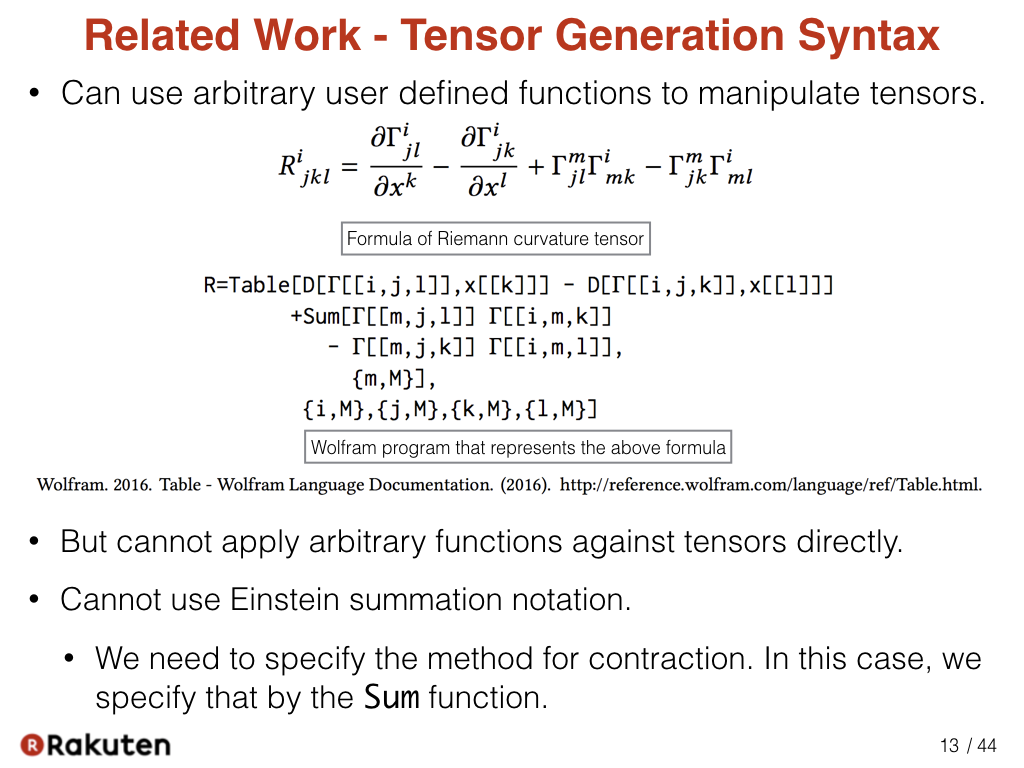In the second method, we describe the computation of the tensor using syntax such as the Table expression of the Wolfram language. The table expression is used to generate an n-dimensional array. It takes an expression that describes each component and the ranges of the index after that. This method has the advantage that we can use an arbitrary function defined for scalar values for tensor operations, such as the differential function “D”. However, this method has the disadvantage that we cannot directly apply user-defined functions to tensor arguments. As the result, the description of a program becomes more complicated than the description directly using index notation.

### #14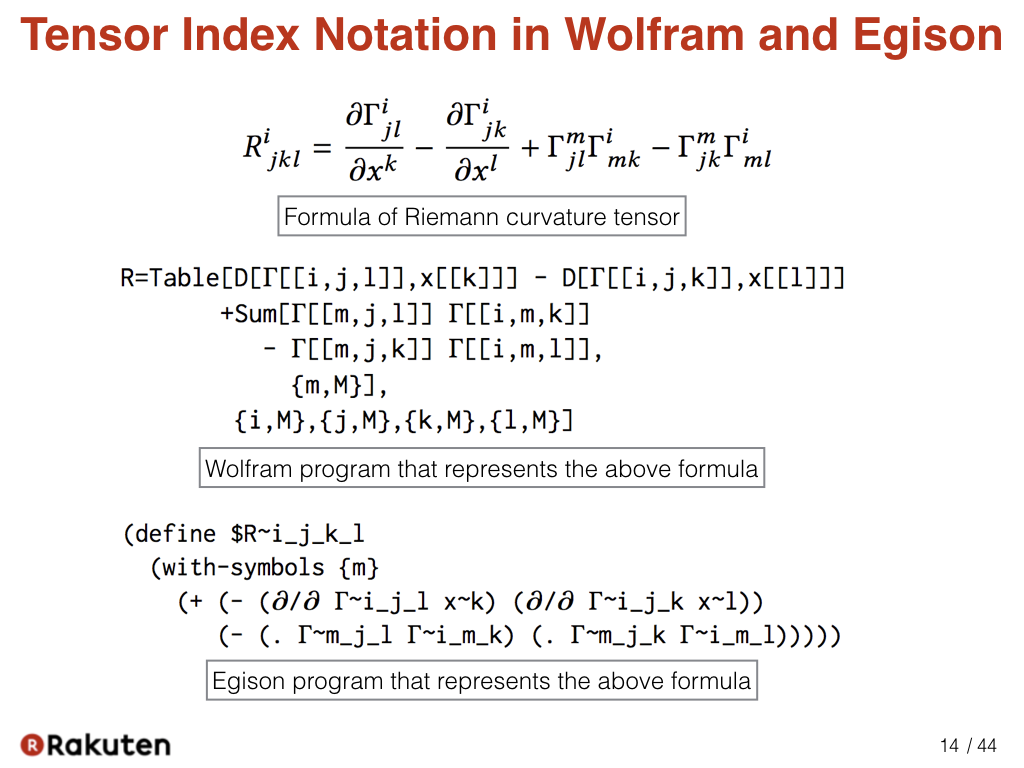These are programs that represent the same formula in Wolfram and Egison.

Please note that a double loop consisting of the Table and Sum expressions appears in the program in the Wolfram language, whereas the program in Egison is flat, similar to the mathematical expression. This is achieved by using tensor index notation in the program.

In particular, the reason that the loop structure by the Sum expression in the Wolfram language does not appear in Egison to express these terms (third and forth term) is that the ”.” function in Egison can handle Einstein summation notation.

Please also pay particular attention to that the Egison program “(∂/∂ Γ~i_j_k x~l)” expressing this term. In the Wolfram language, the differential function “D” is applied to each tensor component, but the differential function “∂/∂” is applied directly to the tensors in Egison.

From the above discussion, we can conclude that Egison expresses mathematical expressions more directly than the Wolfram language, though there is little difference in the number of lines in the programs.

### #15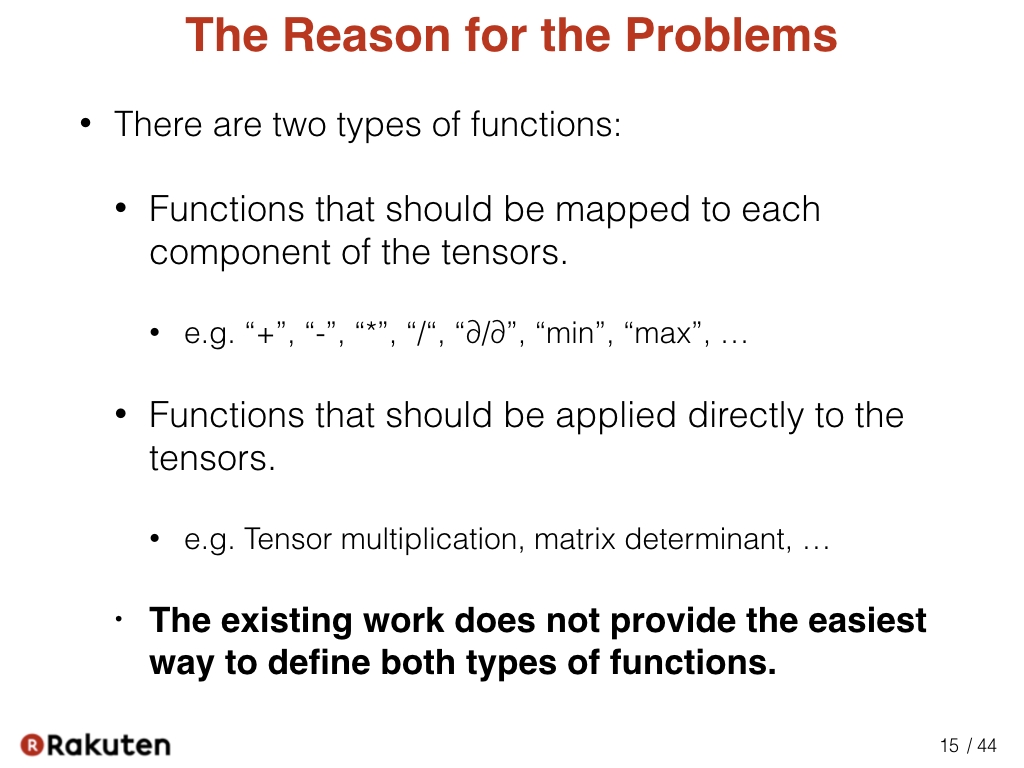This is the reason for the problems. We have to recognize there are two types of functions.

Functions that should be mapped to each component of the tensors, such as “+”, “-”, and ∂/∂”. And functions that should be applied directly to the tensors, such as tensor multiplication, and matrix determinant. In my proposal, these two types of functions are distinguished and called scalar functions and tensor functions.

The reason for the problems in the existing work is they do not provide the easiest way to define both types of functions.

### #16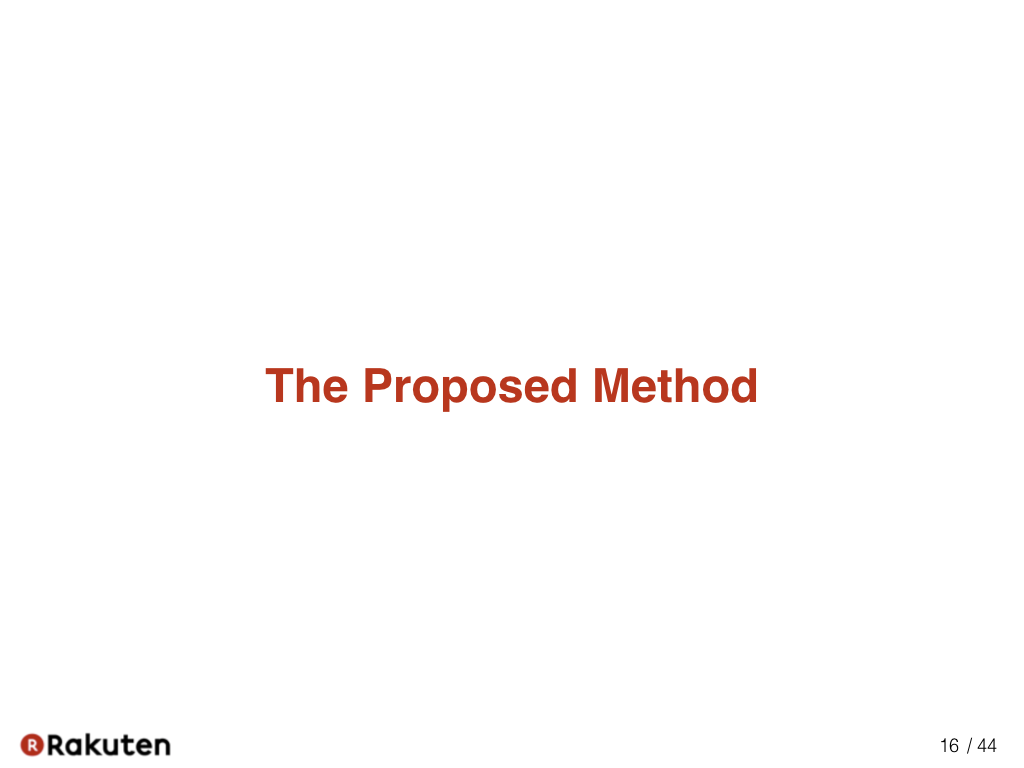Now, let me show my solution.

### #17The novelty of my proposal is that it introduces two types of parameters, scalar parameters and tensor parameters. It enables us to apply arbitrary user-defined functions to tensor arguments using index notation without an additional description.

The function name “min” is also prefixed by the “$” sign, but just as a convention of Egison. Thus it can be ignored. ### #19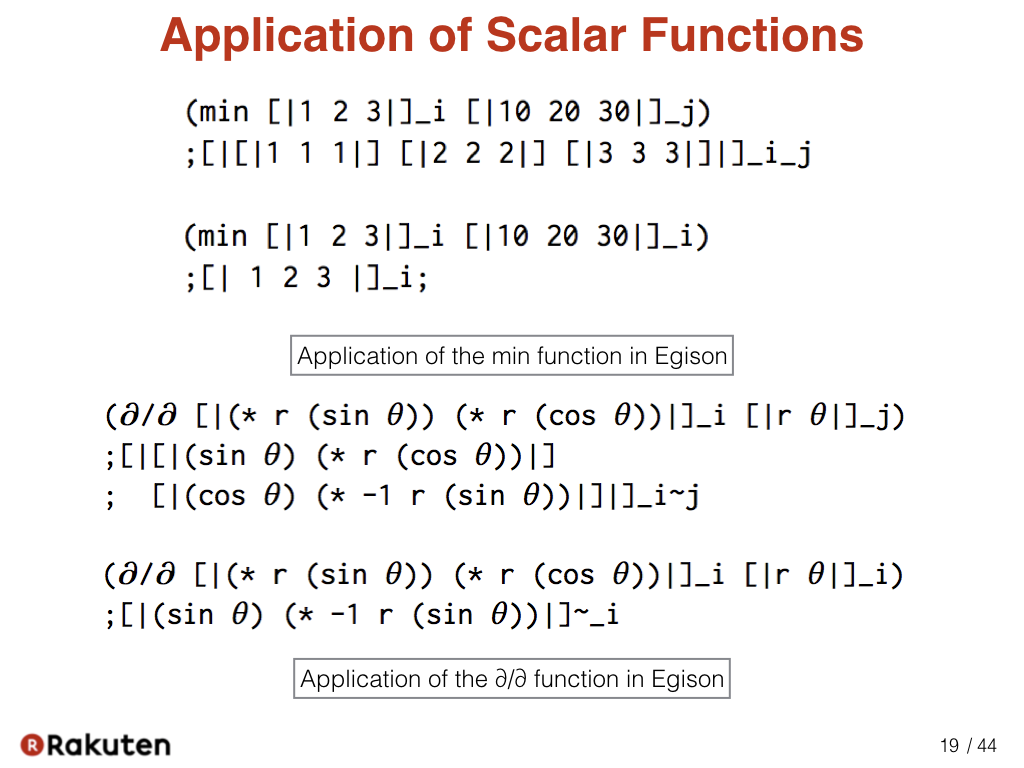In Egison, we can apply the min function as the these samples (above). Here, we also shows the application of partial derivative as the sample of another scalar functions. ### #20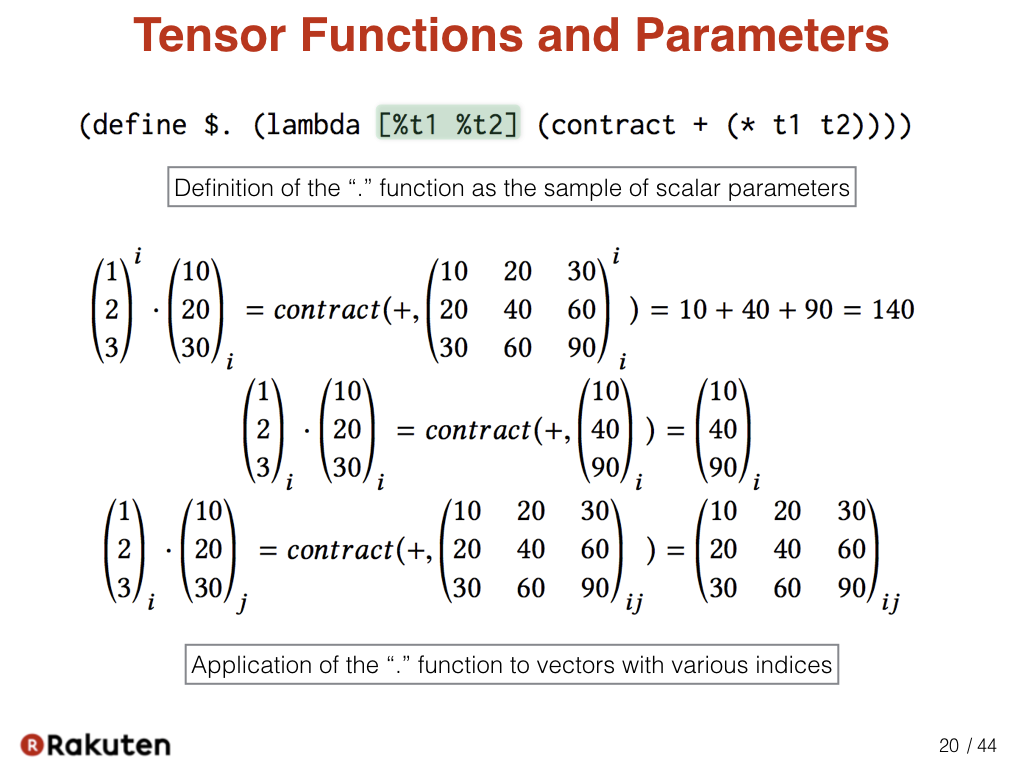This is the definition of the ”.” function as a sample of tensor parameters. “.” is a function for multiplying tensors. The “%” sign is prepended to the beginning of the parameters of the “.” function. It means the parameters of the “.” function are tensor parameters. As with ordinary functions, the function treats the tensor argument as a whole when a tensor is provided to a tensor parameter. “+” and “*” in the definition of the “.” function are scalar functions for addition and multiplication. The contract is a primitive function to contract a tensor that has pairs of a superscript and subscript with identical symbols. Here, we can see the example for calculating the inner product of two vectors using the “.” function. We can use the “.” function for any kind of tensor multiplication such as tensor product and matrix multiplication as well as inner product. ### #21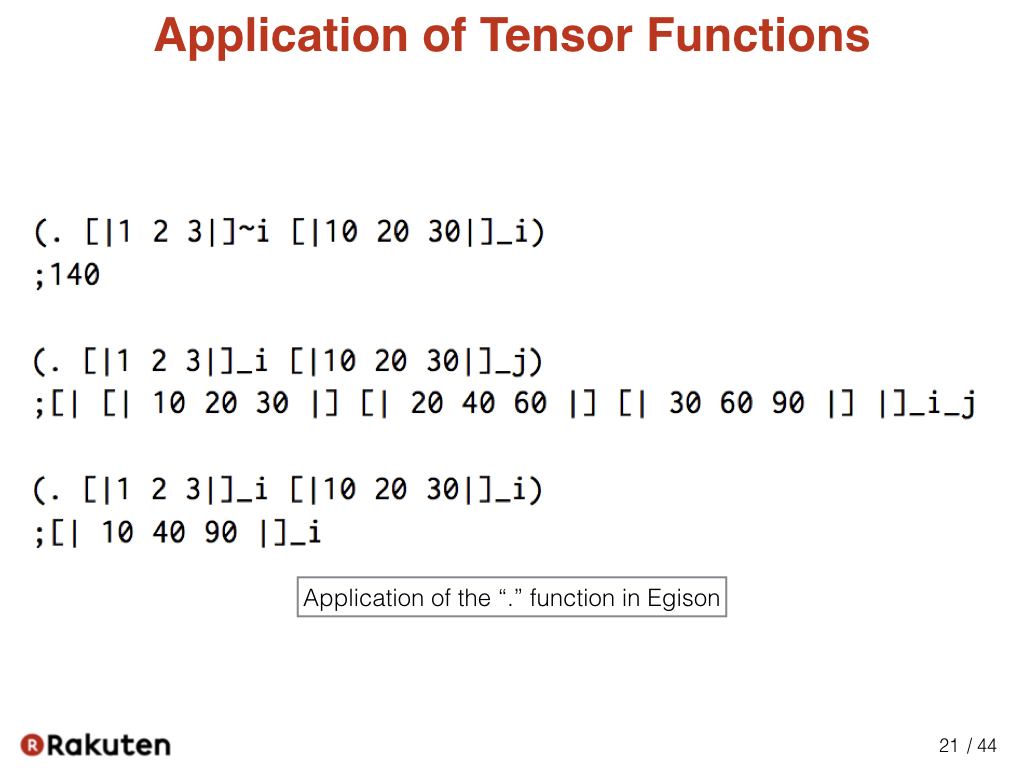In Egison, we can apply the “.” function that way. ### #22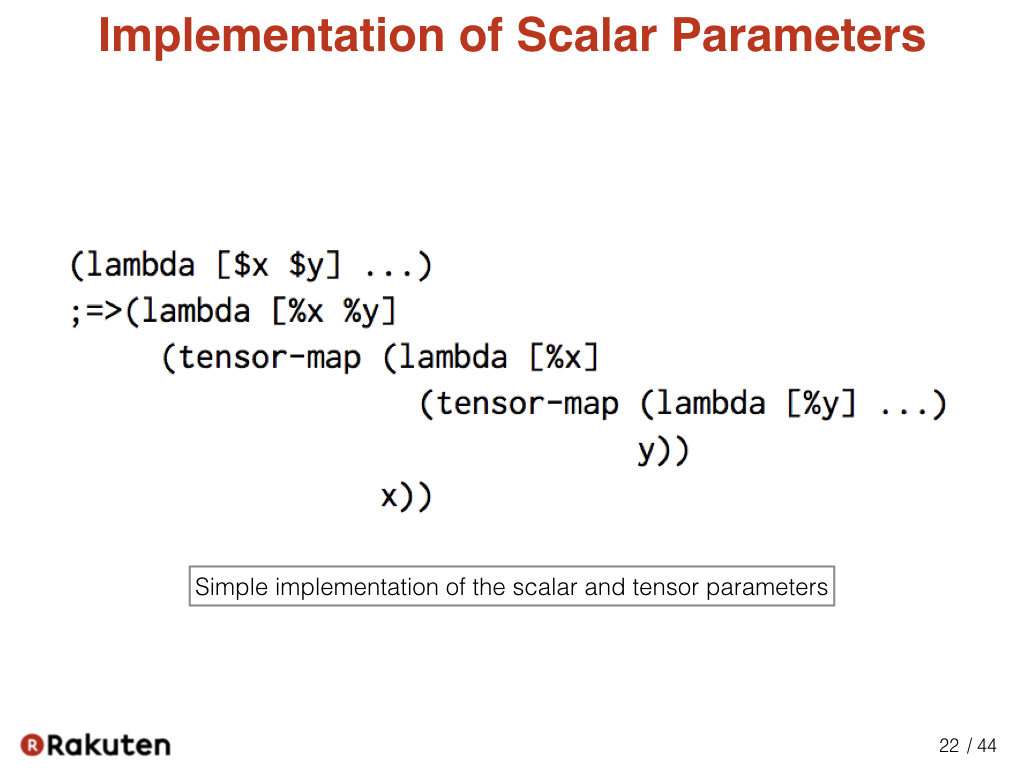A function with scalar parameters is converted to a function only with tensor parameters by using the tensor-map function as follows. That way, we can implement scalar parameters. ### #23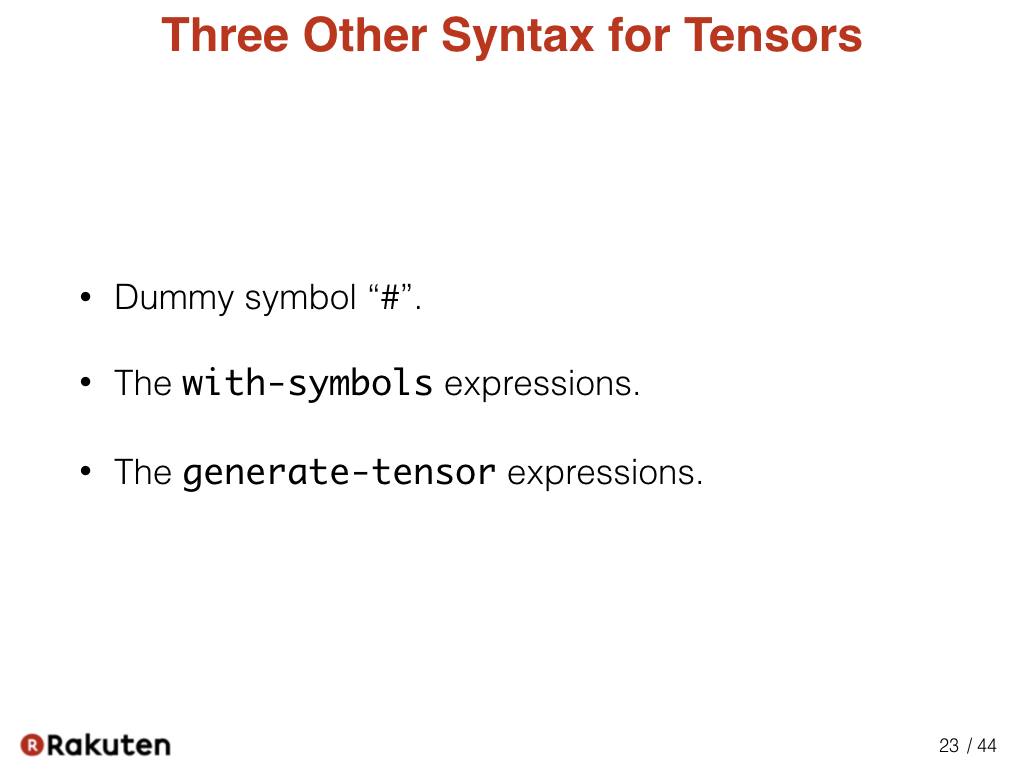Now, let me show three other syntax for tensors. ### #24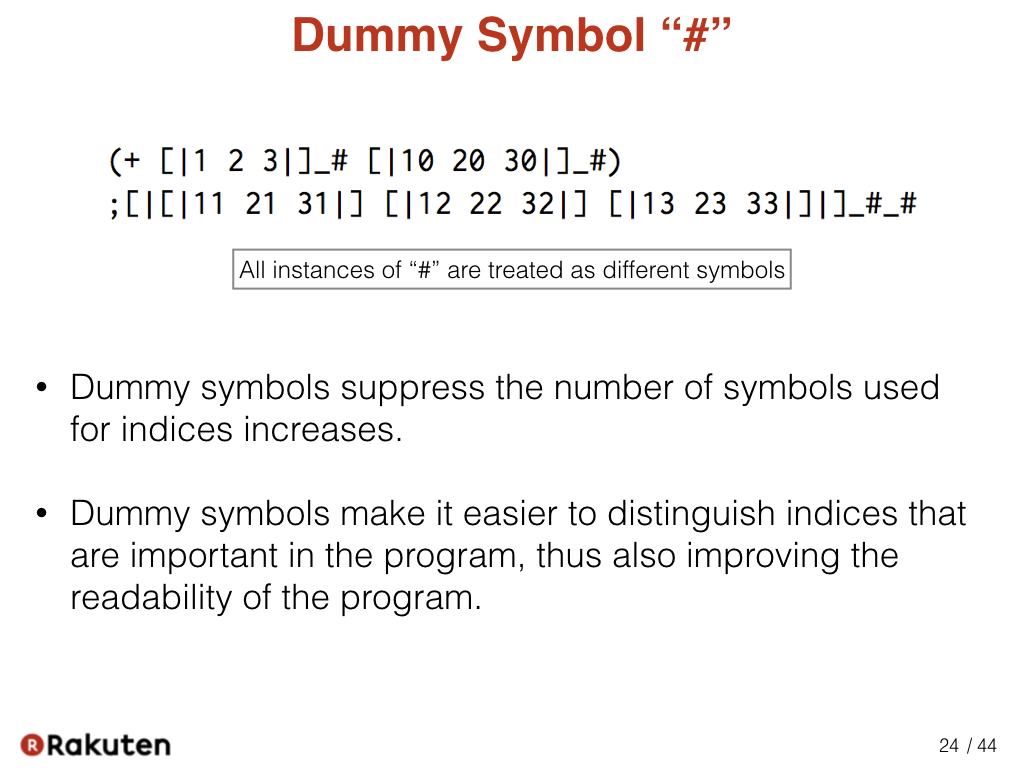We represent a dummy symbol with the “#” sign. All instances of “#” are treated as different symbols. Dummy symbols suppress the number of symbols used for index. Dummy symbols make it easier to distinguish the index that are important in the program, thus also improving the readability of the program. ### #25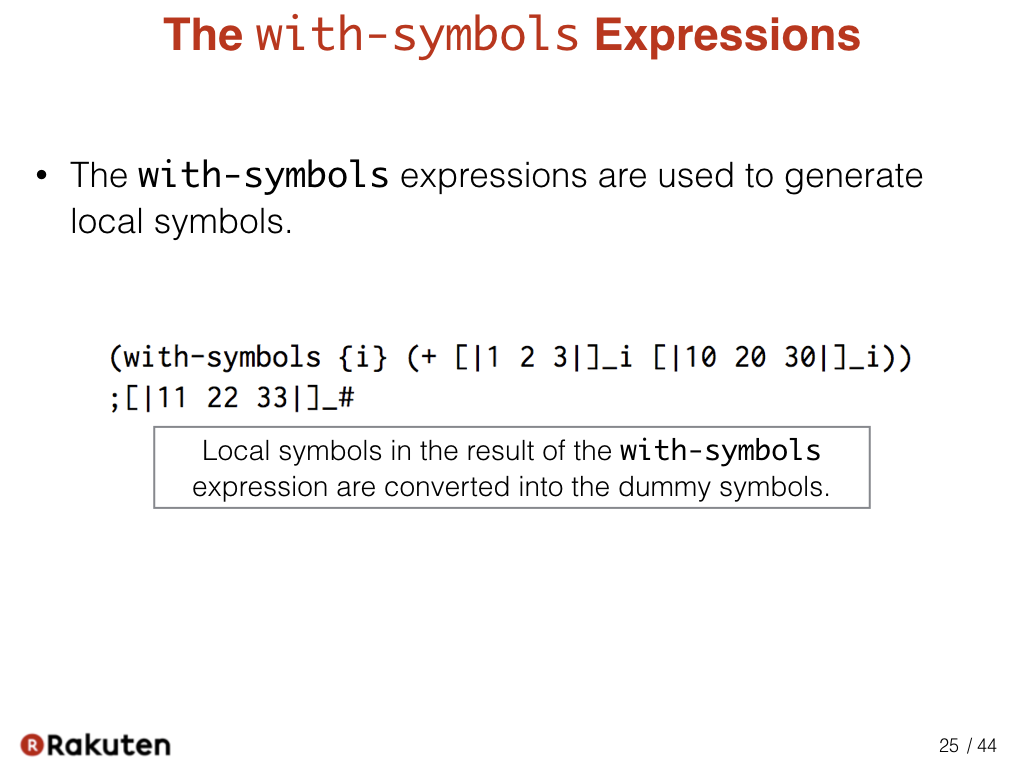The with-symbols expressions are used to generate local symbols. Local symbols in the result of the with-symbols expression are converted into the dummy symbols as in this sample. ### #26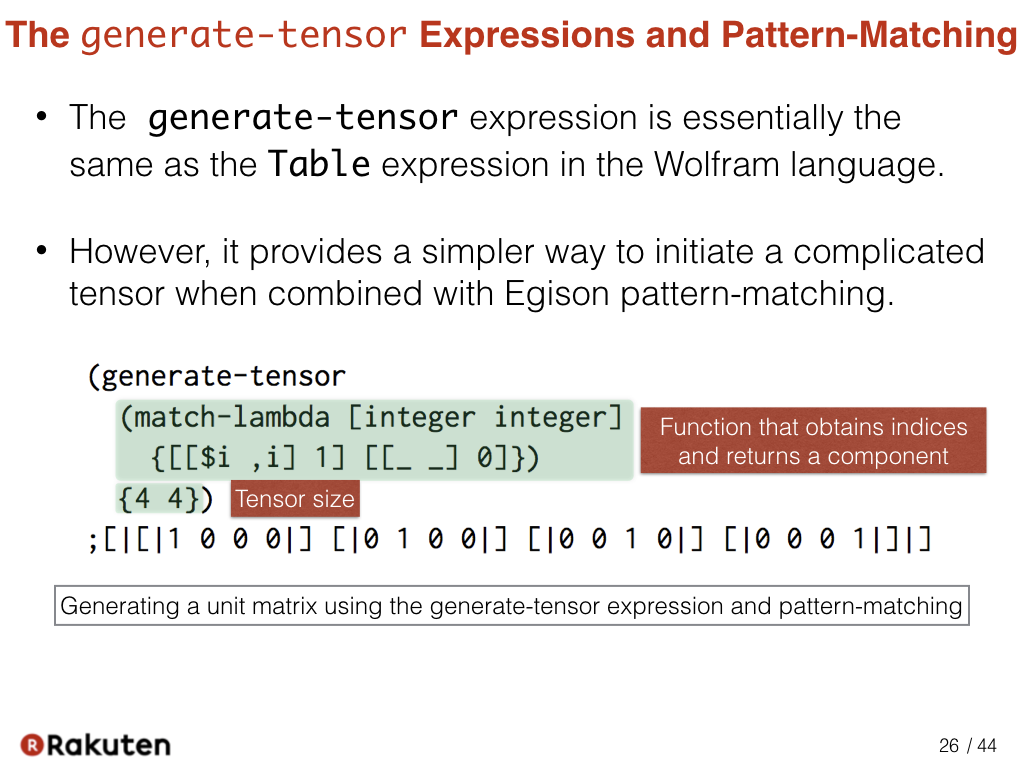The generate-tensor expression is essentially the same as the Table expression in the Wolfram language. However, it provides a simpler way to initiate a complicated tensor when combined with Egison pattern-matching. This sample program generates a unit matrix using the generate-tensor expression and pattern-matching. The generate-tensor expression take a function that obtains indices, and the tensor size. ### #27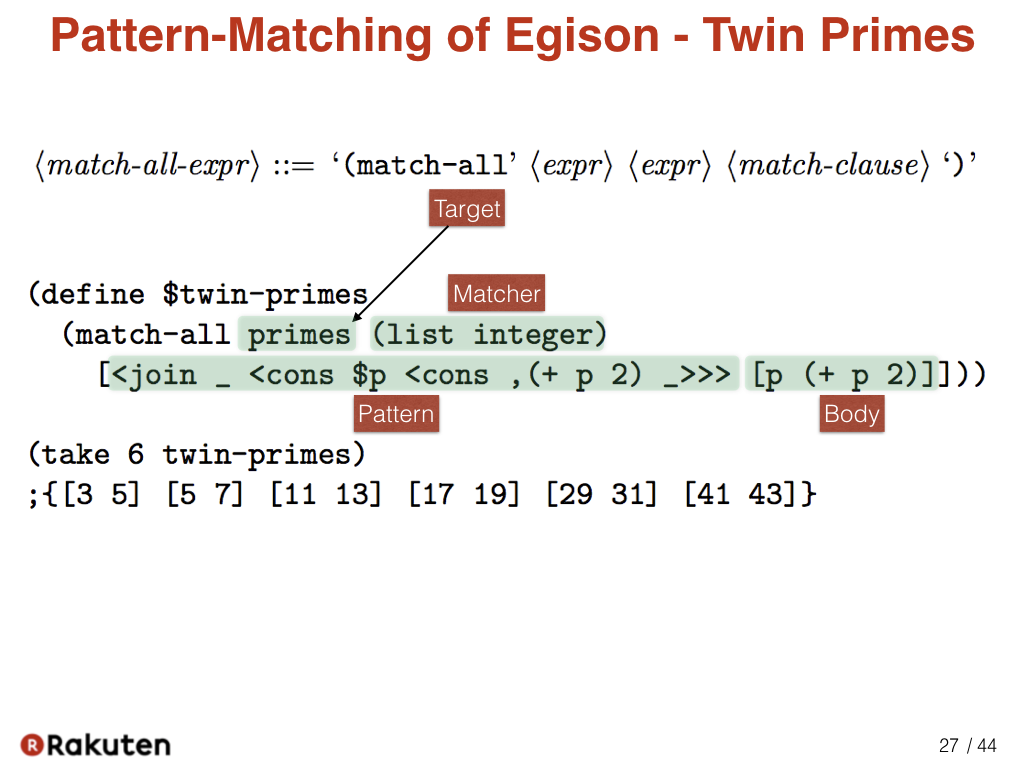Next, let me show a sample program of Egison pattern-matching. This is a sample program of non-linear pattern-matching with multiple results. Non-linear patterns are patterns that allow multiple occurrences of same variables in a pattern. Non-linear pattern-matching is one of the special feature of Egison pattern-matching. This is a sample of a non-linear pattern. The primes in this program contains the infinite list of prime numbers. We match against that as a list of integer. This is the pattern to match a twin-prime. “join _” discards head elements from the list of primes. With “cons$p”, we extract one prime and bind it to the variable p. “cons ,(+ p 2)” matches if the next prime after “p” is “p + 2”. Thus, the pattern matches with a twin-prime. In the body, we return “p” and “p+2”. The match-all expression returns all successful results of pattern-matching. Thus, it enumerates all twin primes.

Here, we extract the first 6 twin-primes.

### #28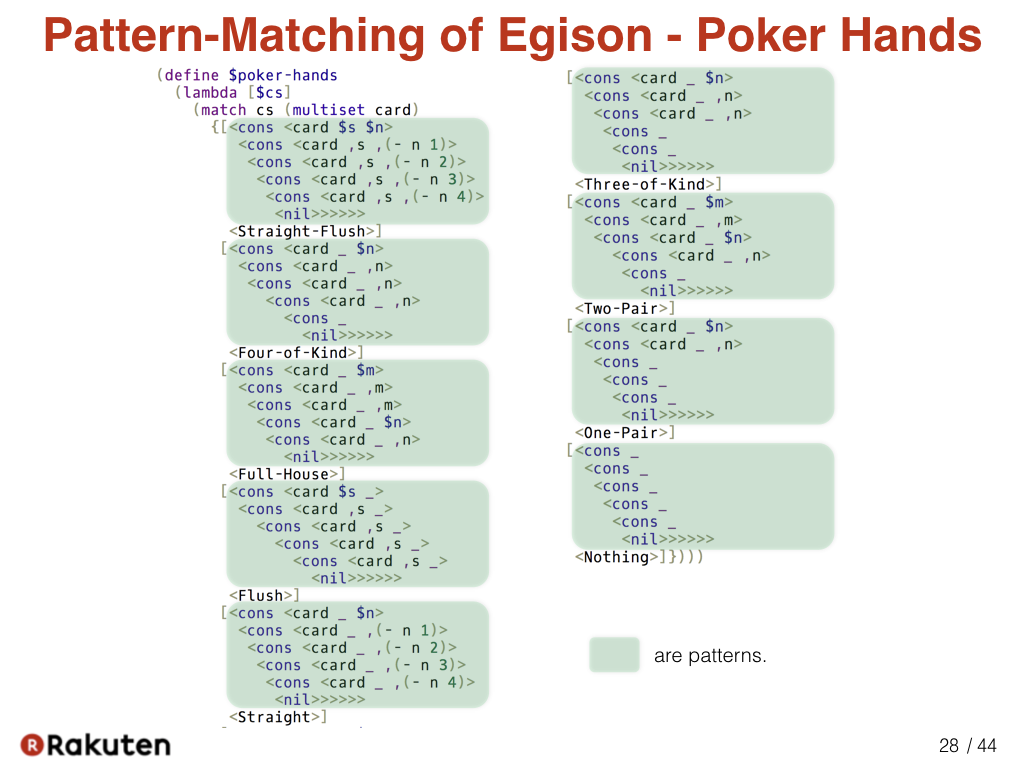The speciality of Egison pattern-matching is that we can represent pattern-matching against wider range of data types. For example, we can represent pattern-matching against multisets as this sample. We can write a program to determine poker hands very concisely if we combine non-linear pattern-matching and pattern-matching against multisets. Actually, the method for pattern-matching against multisets is defined in Egison. So users can define it by themselves.

### #29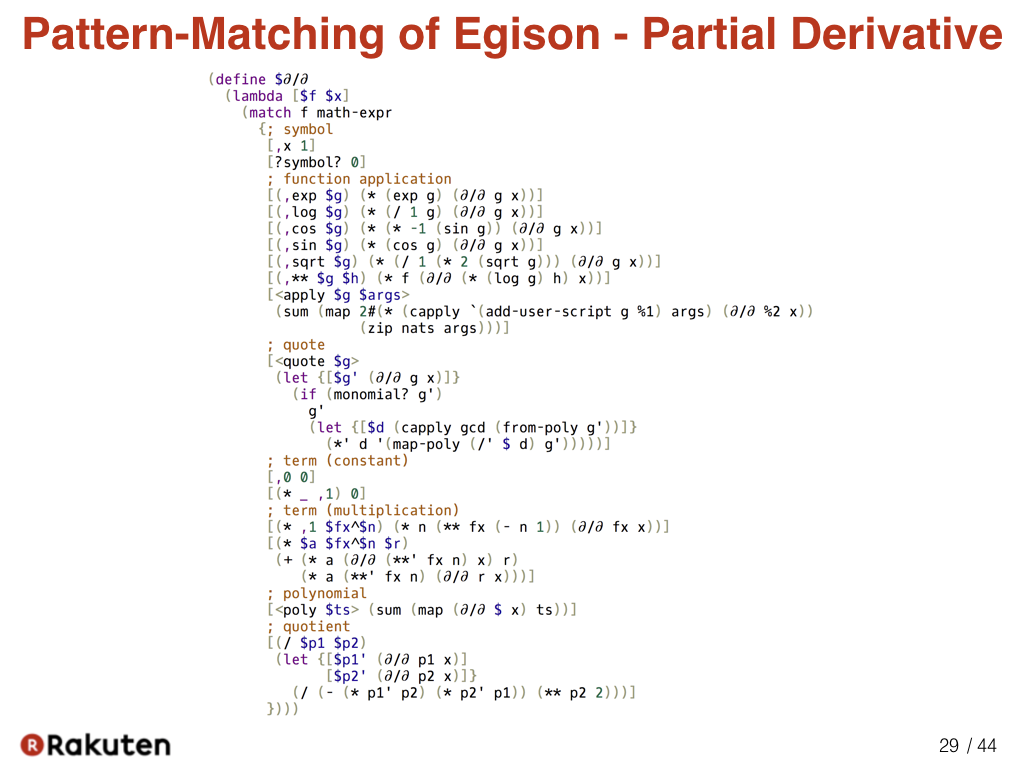Here is a program that defines the symbolic partial derivatives in Egison. Pattern-matching against mathematical expressions is utilized to define that. A mathematical expression is a multiset of terms. A term is a multiset of factors. So pattern-matching against mathematical expression can be described concisely in Egison.

### #30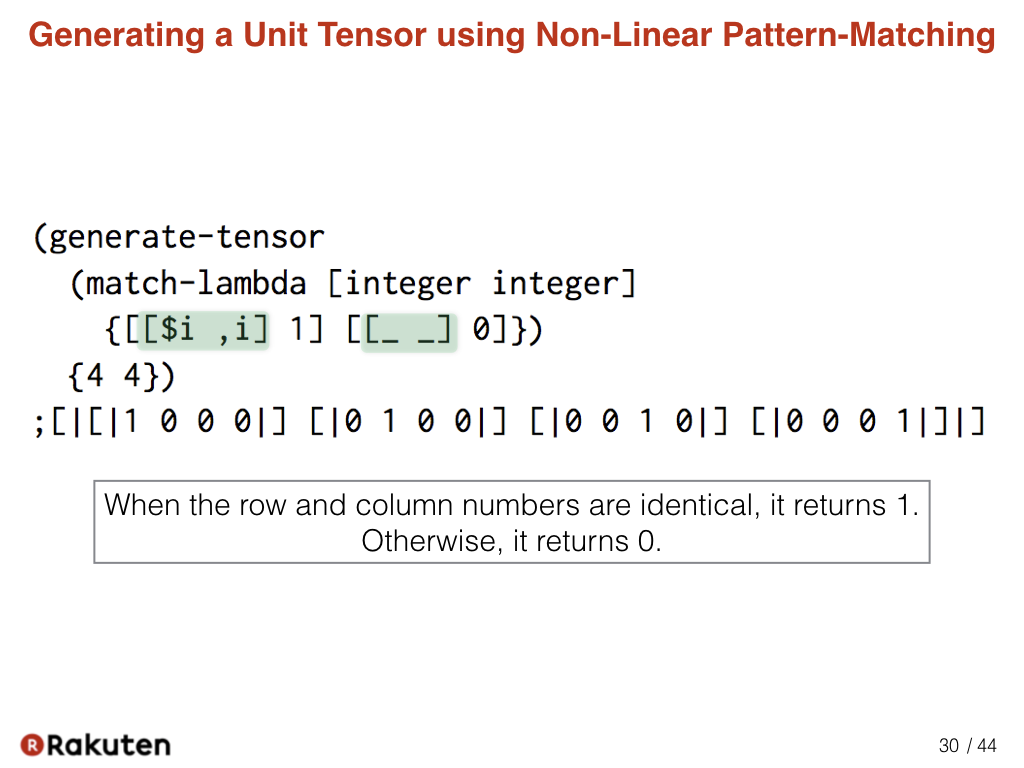This sample code generates a unit matrix using the generate-tensor expression and pattern-matching. When the row and column numbers are identical, it returns 1. Otherwise, it returns 0. Non-linear pattern-matching is used to represent that.

### #31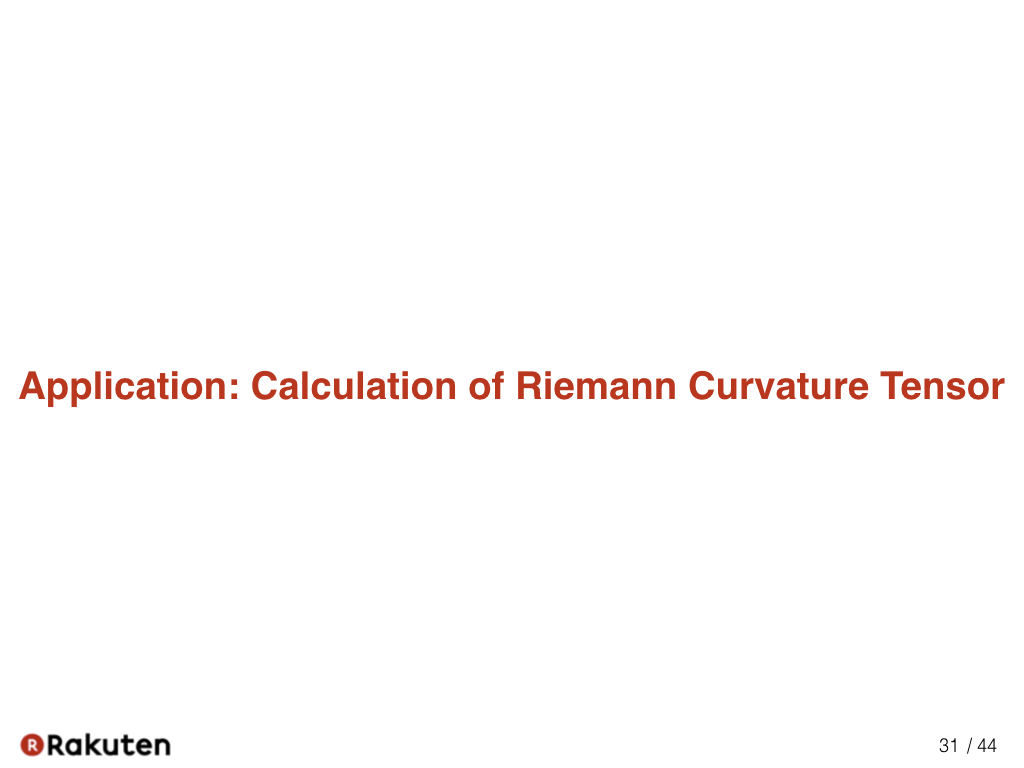Now, let me show you how we can describe the calculation of the Riemannian curvature tensor as an sample application of the proposed method.

### #32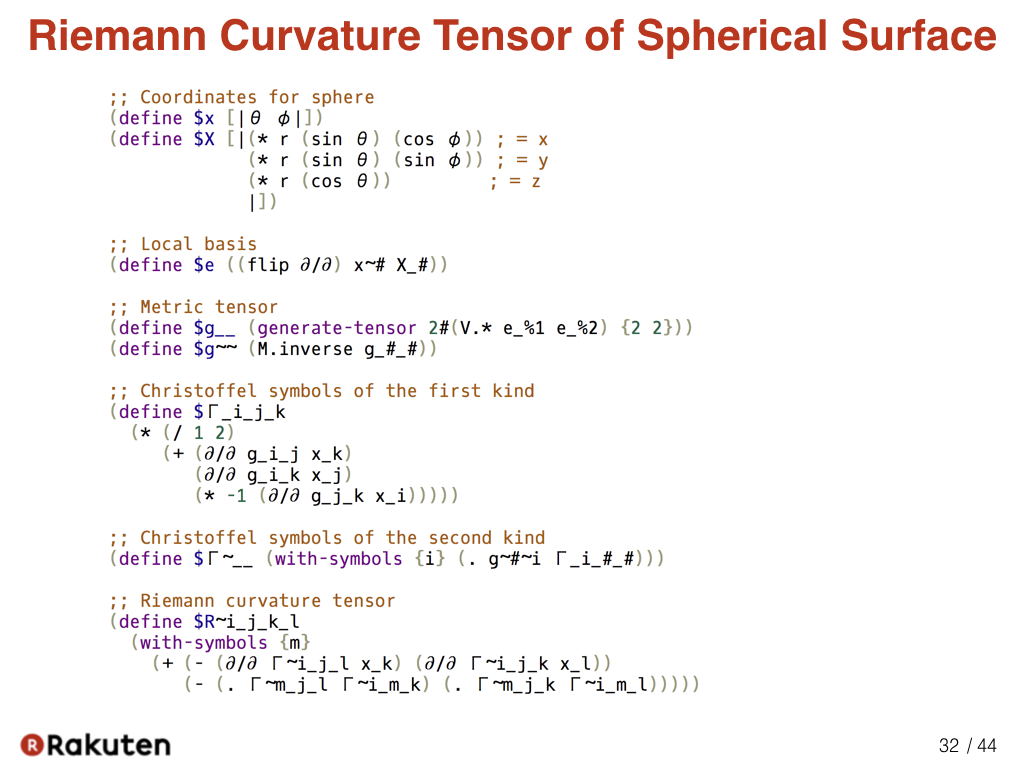Actually, we can write a program to calculate the Riemann curvature tensor of the surface of a sphere in one slide.

### #33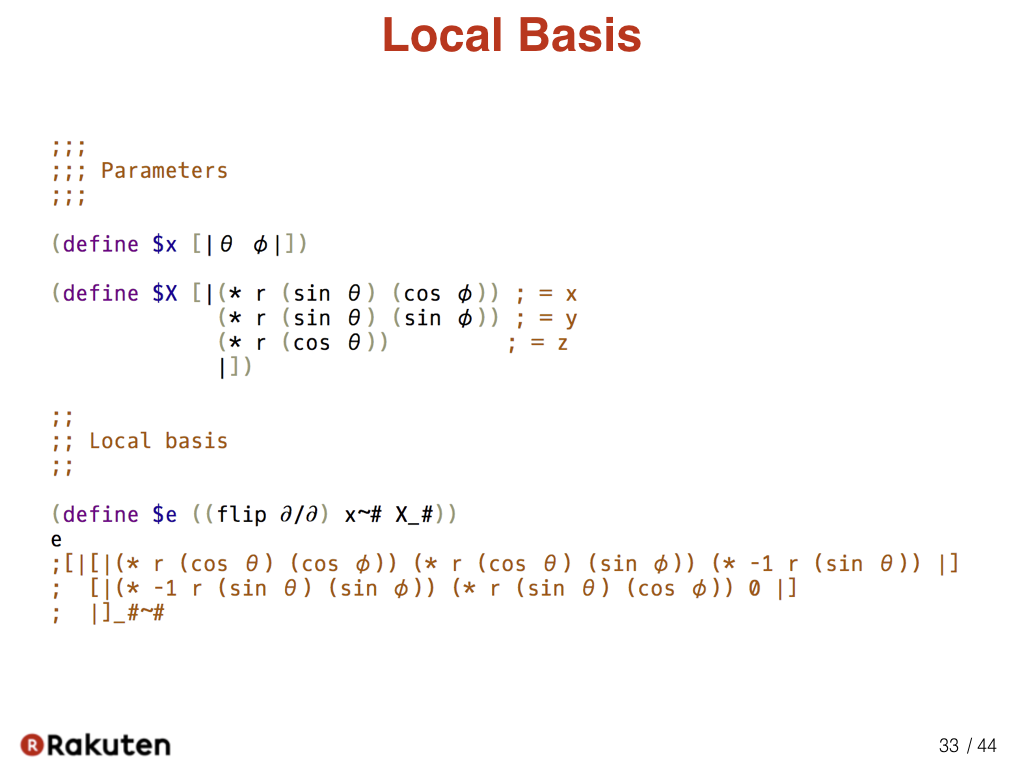In this part, we set the parameters and calculate the local basis.

### #34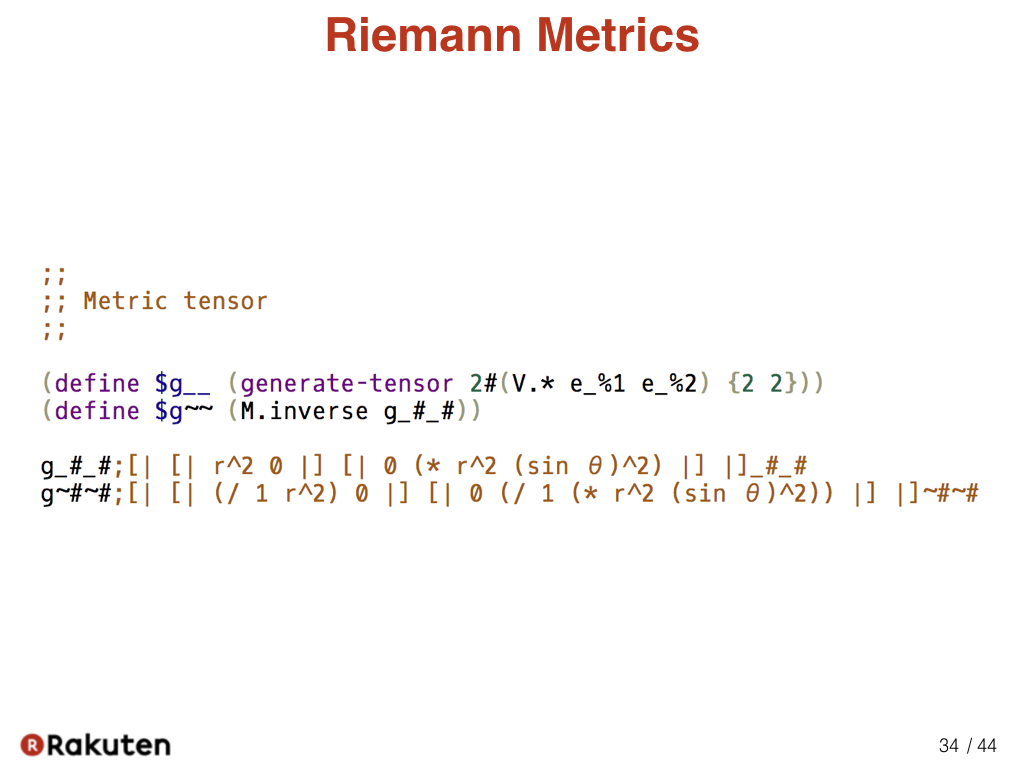In this code, we calculate Riemann metrics of the surface of a sphere.

In Egison, we can specify the type of indices in the variable name when binding a tensor to a variable. For example, we can bind different tensors to “g__” and “g~~”.

### #35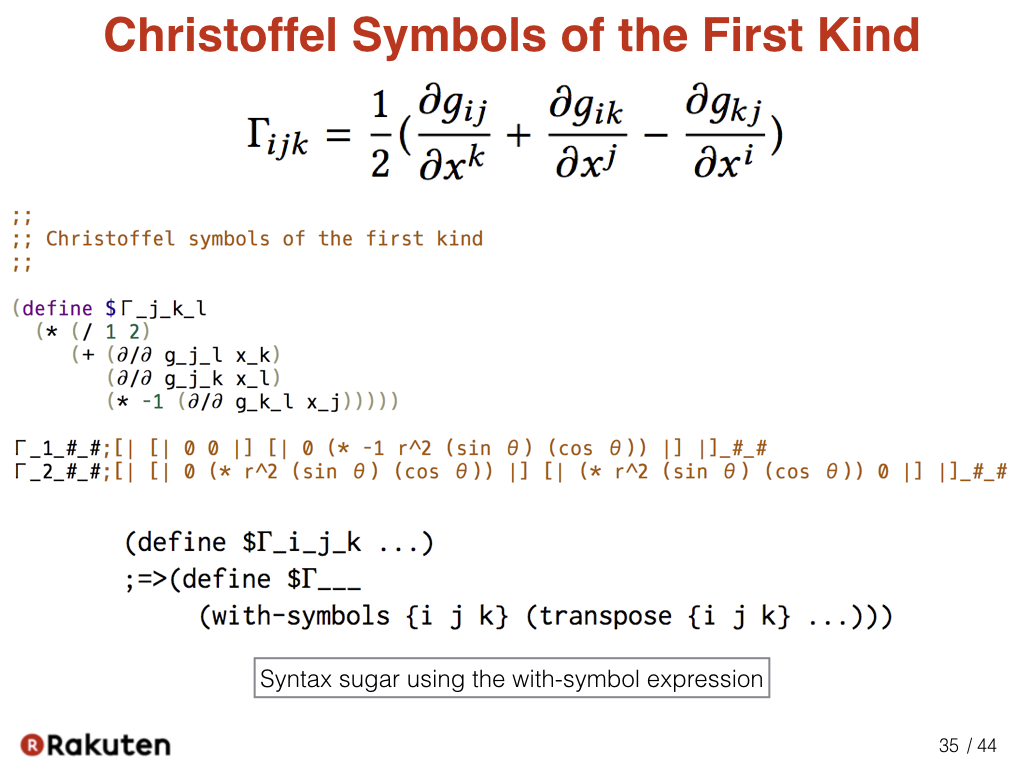Here, we calculate Christoffel symbols of the first kind. Tensor index notation is effectively used to represent the formula.

Here, the tensor is bound to a variable with symbolic indices such as “Γ_i_j_k” It is automatically desugared as follows. This syntactic sugar renders a program closer to the mathematical expression. The transpose is a function for transposing the tensor in the second argument as specified in the first argument.

### #36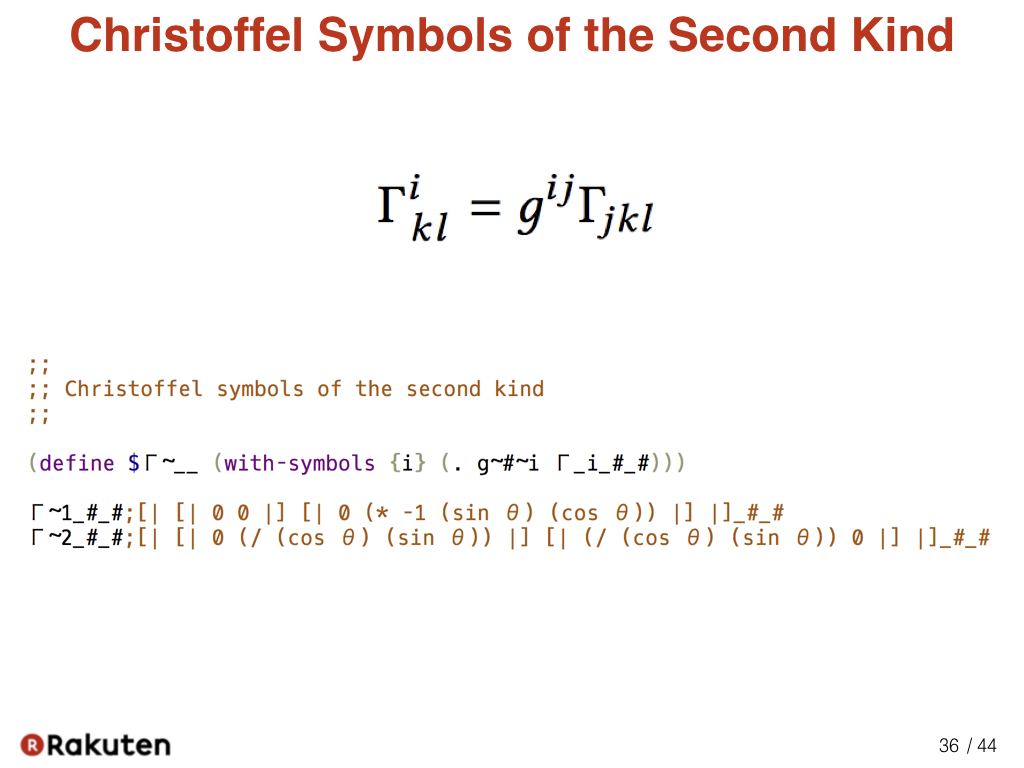Here, we calculate Christoffel symbols of the second kind. The formula is directly represented.

### #37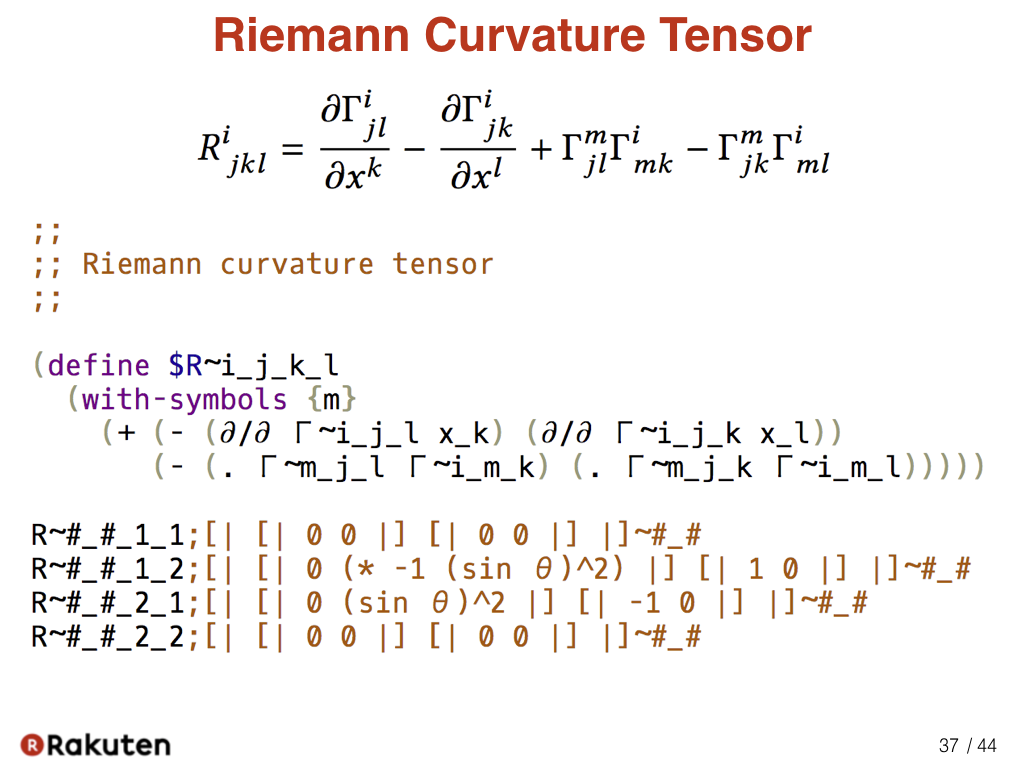This is a part of the program that is representing the formula of Riemann curvature tensor.

### #38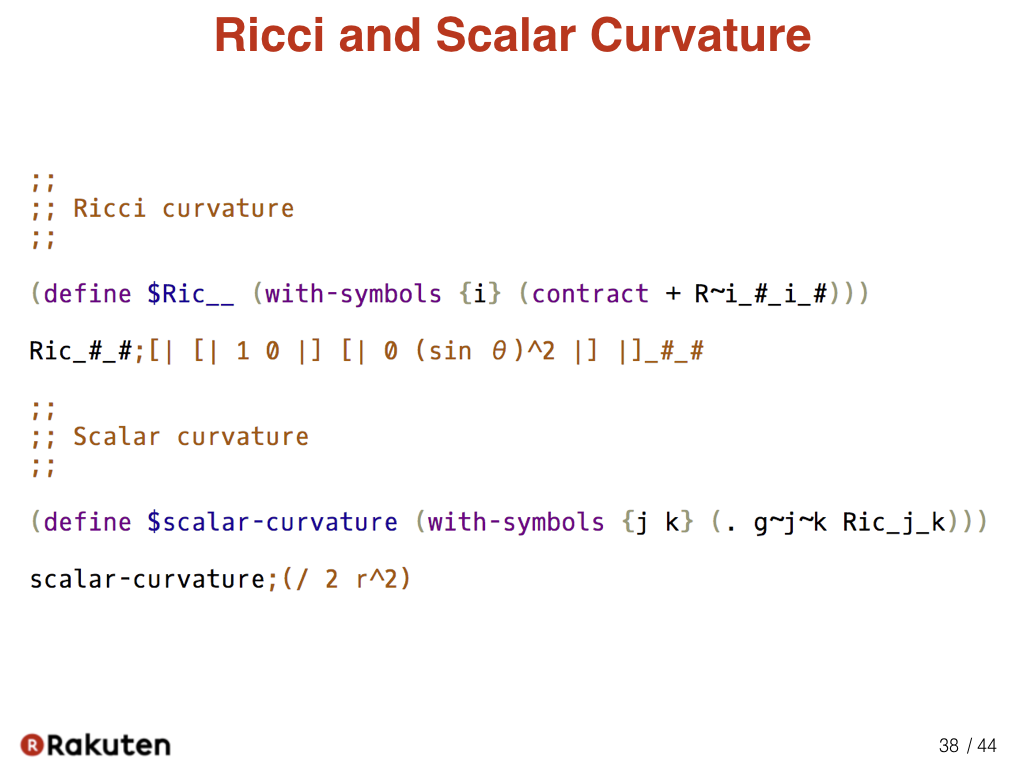We can also describe the calculation of the Ricci and scalar curvature concisely.

### #39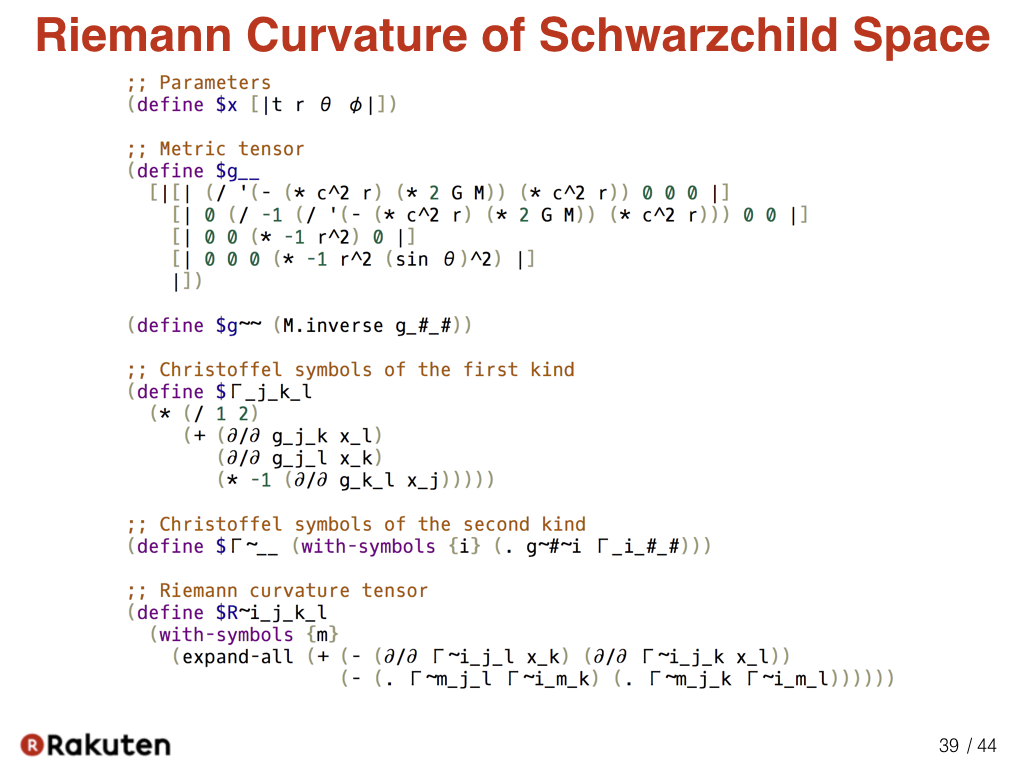Here is a program that describe the calculation of the general theory of relativity. This program calculates the Riemann curvature tensor of Schwazchild space, the space-time where there is only one star in the origin. If we analyze the result of this program, we can see the reason of gravity geometrically.

Actually, I found the proposed method when I was trying to implement this calculation as concisely as possible extending Egison.

### #40Finally, let me explain my future plans.

### #41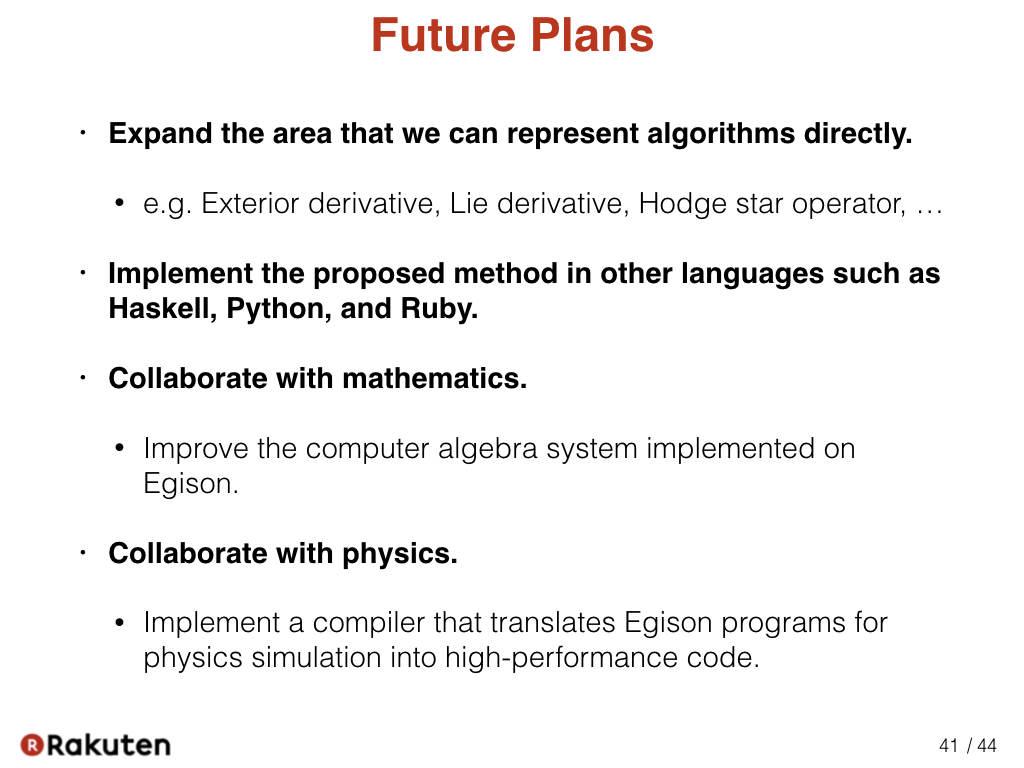First of all, I am trying to expand the area that we can represent algorithms directly. For example, I am currently researching a method for describing exterior derivative, Lie derivative, and Hodge star operation concisely. In addition to index notation, there are still many notations in mathematics that are useful, but not yet introduced into programming. There might also be notations that mathematicians have not discovered yet, but that describe the formulas of existing theories more concisely.

Second, I am planning to implement the proposed method in other languages. I think it is an interesting research topic to think about how to import the proposed method into existing programming languages naturally. For example, in a static type system, whether the parameter of a function is a scalar or tensor parameter can be specified when specifying the type of the function.

Third, I am trying to collaborate with mathematics. For that, I have to improve the computer algebra system implemented on Egison.

Fourth, I am collaborating with physics. It is of substantial significance to import this method into programming languages that have a compiler that generates high-performance code for physical simulation. For example, incorporating this method would enable us to describe physical simulation using not only the Cartesian coordinate system but also more general coordinate systems such as the polar and spherical coordinate system in simple programs.

### #42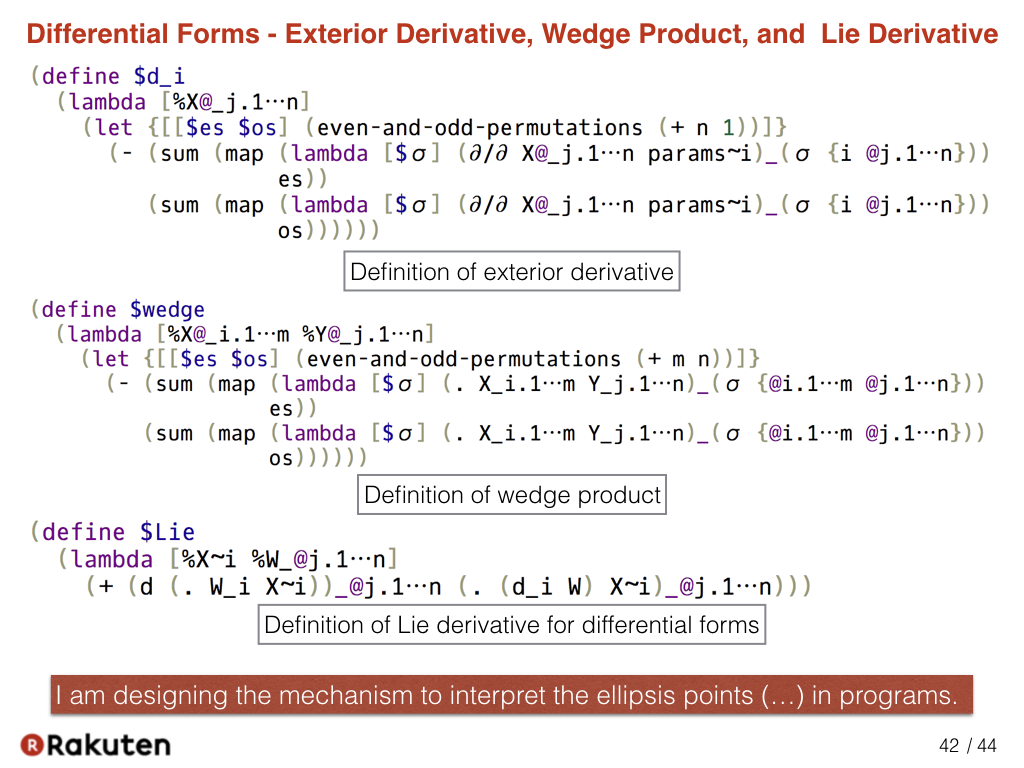Here is the current design that represents the definition of exterior derivative, wedge product, and Lie derivative. In order to describe them, we need to represent the ellipsis points, three dots, in programs. Currently, I am trying to design the best method to interpret the ellipsis points (…) in programs.

### #43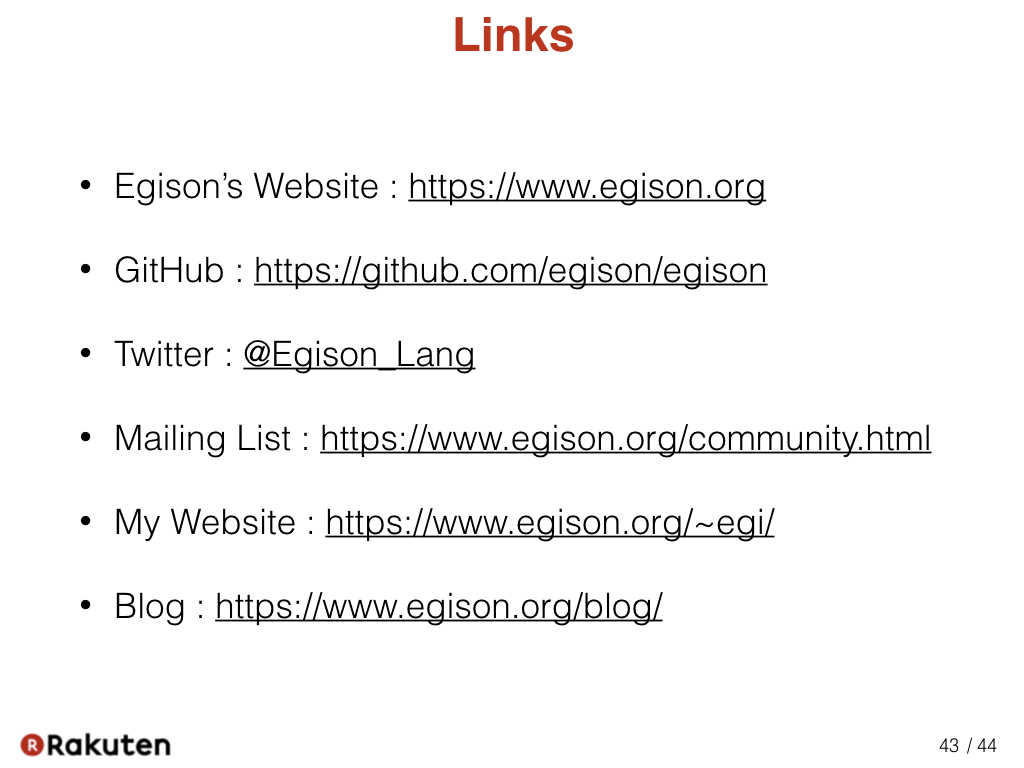These are links for the further information on Egison. Please visit them.

### #44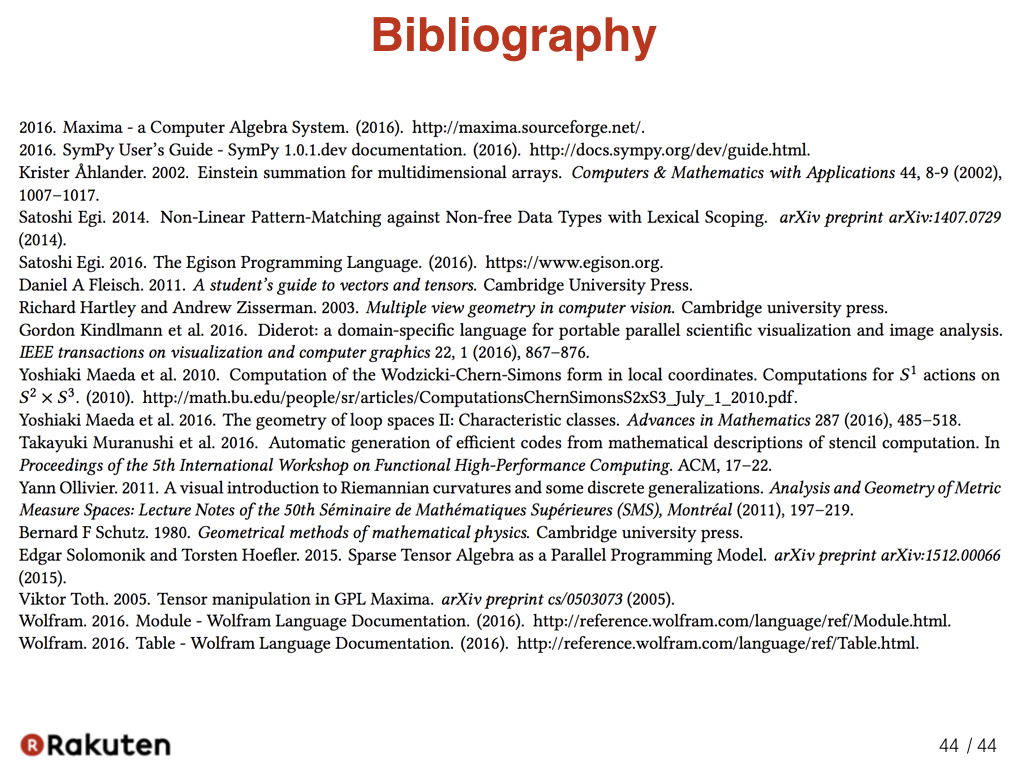That is today’s topic. Thank you very much for your attention. I am happy to hear for your questions now.

### Recent Tweets

This website in other langauge: English, 日本語